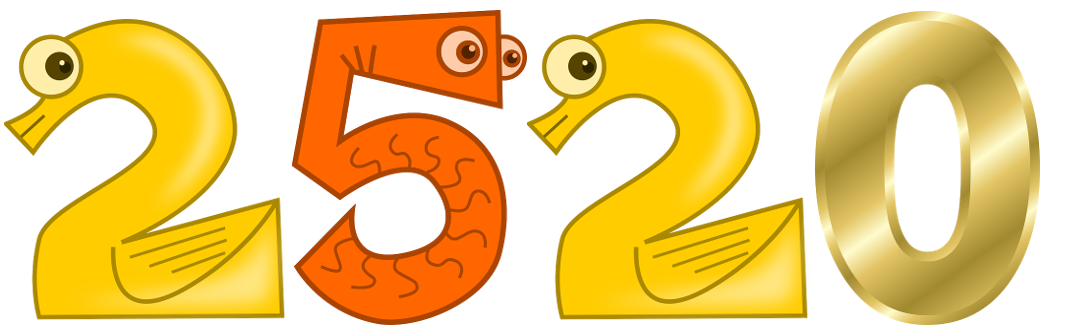# The number 2520 – by Sri Srinivasa RamanujanIn mathematics, no number can be divided by all the numbers from 1 to 10,

But this one number is very strange, all the mathematicians in the world Shocked.

This number was discovered by Indian mathematicians with their unwavering intelligence.

See this number 2520.

It seems to be one of many numbers, But in reality it is not, it is a number that has surprised many mathematicians around the world.

This number can be divided by any number from 1 to 10.

Whether even or odd, This number can be divided by any number from 1 to 10.  The rest is zero.

It sounds like really amazing and impossible numbers.  Now look at the next table.

2520 ÷ 1 = 2520

2520 ÷ 2 = 1260

2520 ÷ 3 = 840

2520 ÷ 4 = 630

2520 ÷ 5 = 504

2520 ÷ 6 = 420

2520 ÷ 7 = 360

2520 ÷ 8 = 315

2520 ÷ 9 = 280

2520 ÷ 10 = 252

The secret of the number 2520 is hidden in the multiplication of [7 × 30 × 12].

With regard to the Indian Hindu year, the riddle of this 2520 number is solved, It is the coefficient of this number.

Days of the week (7),

Days of the month (30)

And months in a year (12)

[7 × 30 × 12 = 2520] This is the characteristic and dominance of time.

The great sage who discovered it was Sri Srinivasa Ramanujan

ಸಂಗ್ರಹ